# How to Count Dates of Current Month in Excel Range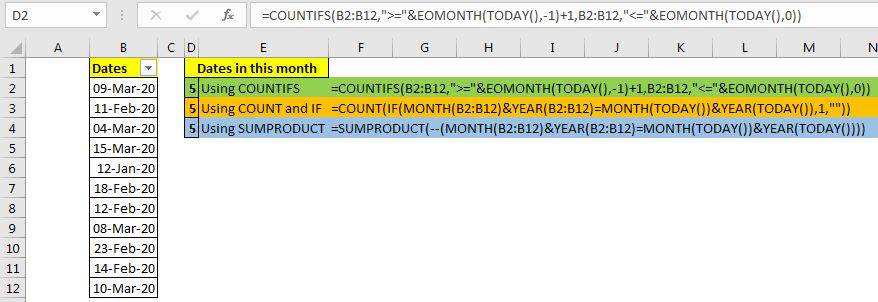Let's say you have to manage meeting dates in an Excel file. Now you want to how many dates (meetings) are there in the current month, next month or the previous month. You will usually use filters to do so. But that's not a solution.

We can count the number of dates in current from an excel range using one formula. It will update automatically and you will not need to use filters for counting dates anymore.

There are three formulas you can use. They are based on:

1. Using COUNTIFS with EOMONTH and TODAY function
2. Using COUNT and IF with MONTH and TODAY function
3. Using SUMPRODUCT with MONTH and TODAY function

Let's check them out one by one.

### Method 1. Count Dates in Current Month Using Excel COUNTIFS with EOMONTH and TODAY function

The Generic formula is

 =COUNTIFS(dates,">="&EOMONTH(TODAY(),-1)+1,dates,"<="&EOMONTH(TODAY(),0))

Dates: It is the range that contains the dates. It can be a range or named range.

EOMONTH(TODAY(),-1)+1: This to get the first date of the current month. You can adjust this section to get the first date of next month or last month by change -1 to 0 and 1 respectively. Increase the number and increase the offset of the month.

EOMONTH(TODAY(),0): This is to get the last date of the current month. If you increase the number in this segment, you get the last dates next month, if you decrease the number you get the last date of previous months.

Use the above two segments to control the month.

Example: Get Meetings Scheduled in the Current Month

Here we have a table that has the dates of the meeting. We need to get the number of dates from the range, planned in the current month.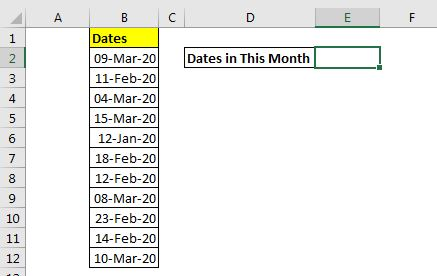Write this formula:

 =COUNTIFS(B2:B12,">="&EOMONTH(TODAY(),-1)+1,B2:B12,"<="&EOMONTH(TODAY(),0))

This returns 5 as there are 5 dates of Feb 2020.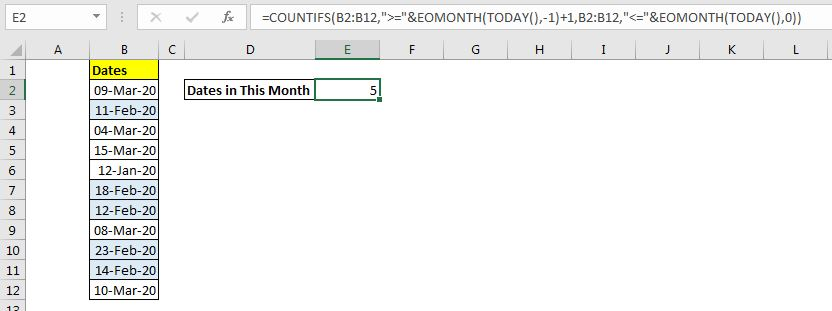How does it work?

Basically, we want to count dates greater than or equal to the first date of the current month and less than or equal to the last day of the current month. So we use the COUNTIF function.

Now we only need to evaluate the first date of the current month and last date of the current month. We do it using the Excel functions EOMONTH and TODAY.

The EOMONTH function returns the last date of the month of the supplied date. We can control the month before and after using the next argument. We provide today's date using the TODAY function. Now EOMONTH(TODAY(),-1)+1 statement returns the first date of the current month (read about EOMONTH function in detail here). EOMONTH(TODAY(),0) returns the last date of the current month.

Eventually, the formula becomes a simple COUNTIFS formula.

 =COUNTIFS(B2:B12,">="&43862,B2:B12,"<="&43890)

This formula returns the count dates of the current month in range B2:B12.

### Method 2. Count Dates in Current Month Using COUNT and IF with MONTH, YEAR and TODAY function

If you don't want to use the EOMONTH function then you can use this formula to count the dates that occur in the current month.

Generic Formula:

 =COUNT(IF(MONTH(dates)&YEAR(dates)=MONTH(TODAY())&YEAR(TODAY()),1,""))

Dates: It is the range that contains the dates. It can be a range or named range.

Let's use this generic formula in the above example. The formula will be:

 =COUNT(IF(MONTH(B2:B12)&YEAR(B2:B12)=MONTH(TODAY())&YEAR(TODAY()),1,""))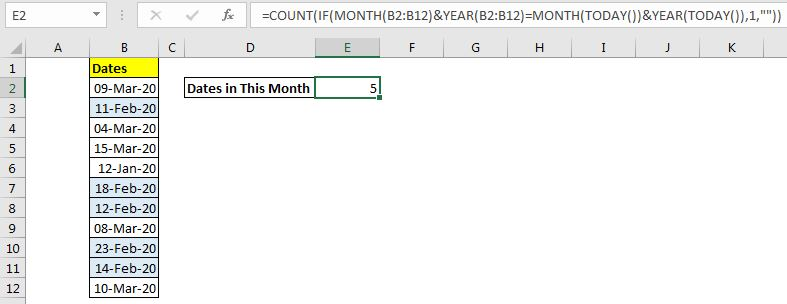How does it work?

The MONTH(B2:B12)&YEAR(B2:B12) part returns an array that contains the month and year of each date. The array will be

{"32020";"22020";"32020";"32020";"12020";"22020";"22020";"32020";"22020";"22020";"32020"}.

The MONTH(TODAY())&YEAR(TODAY()) part creates a string that contains the current month's month number and year. In this example, it is "22020".  This value is compared to each value in the above array using equals (=) sign. This returns an array of TRUE and FALSE.

{FALSE;TRUE;FALSE;FALSE;FALSE;TRUE;TRUE;FALSE;TRUE;TRUE;FALSE}

Now, for each TRUE value, the IF function returns 1 and for FALSE returns nothing ("").

{"";1;"";"";"";1;1;"";1;1;""}

Finally, COUNT function counts the numeric values from array and returns the dates of the current month from the selected range.

### Method 3. Count Dates in Current Month Using SUMPRODUCT with MONTH, YEAR and TODAY function

SUMPRODUCT function is amazing when it comes to counting and summing values with crazy criteria.

In this method, we use boolean logic to count the dates of the current month a range.

 =SUMPRODUCT(--(MONTH(dates)&YEAR(dates)=MONTH(TODAY())&YEAR(TODAY())))

Dates: It is the range that contains the dates. It can be a range or named range.

When we use this function in our example, we get the exact same answer.

 =SUMPRODUCT(--(MONTH(B2:B12)&YEAR(B2:B12)=MONTH(TODAY())&YEAR(TODAY())))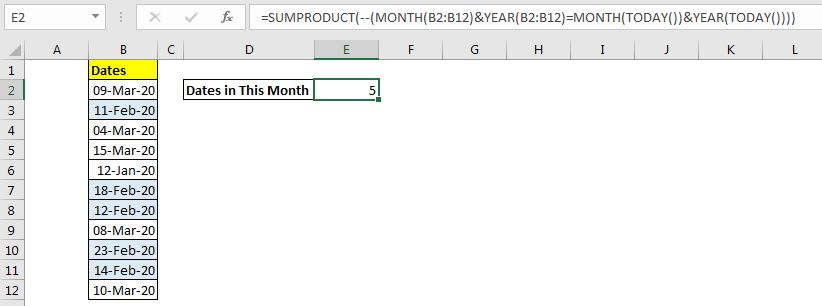How does it work?

(MONTH(B2:B12)&YEAR(B2:B12)=MONTH(TODAY())&YEAR(TODAY())): This segment does the same thing as it does in the COUNT and IF method and returns an array of TRUE and FALSE.

{FALSE;TRUE;FALSE;FALSE;FALSE;TRUE;TRUE;FALSE;TRUE;TRUE;FALSE}

Outside this statement, we have a double negative operator (--) to convert these TRUE and FALSE values into 1s and 0s. Finally, we have the formula simplified to:

SUMPRODUCT({0;1;0;0;0;1;1;0;1;1;0})

The SUMPRODUCT function sums up this array and returns the count of the number dates of the current month in the range.

So yeah guys, these are the ways two count dates of the current month. Choose the one that suits you best. I hope It was explanatory and helpful. If you have any doubts regarding this topic, ask in the comments section below. I will be happy to hear from you.

Related Articles:

How to Countdown Remaining Days in Excel | To count remaining days we use simple subtraction formula in Excel. If you want to count the remaining days from the current date, then we use the TODAY function.

How to Calculate days, months and years | To calculate days, month and year, Excel has three functions called DAY, MONTH and YEAR. These functions get the day, month and year from the given date.

How to Calculate Remaining Days of Month in Excel | To calculate the remaining days of a month, we use the EOMONTH function. We subtract the current date from the date of the end of the month.

Count Birth Dates from range by month Excel| Using function SUMPRODUCT and MONTH. This function will let us know how many birthdates are in a specific month.

Popular Articles:

50 Excel Shortcuts to Increase Your Productivity | Get faster at your task. These 50 shortcuts will make you work even faster on Excel.

How to use Excel VLOOKUP Function| This is one of the most used and popular functions of excel that is used to lookup value from different ranges and sheets.

How to use the Excel COUNTIF Function| Count values with conditions using this amazing function. You don't need to filter your data to count specific value. Countif function is essential to prepare your dashboard.

How to Use the SUMIF Function in Excel | This is another dashboard essential function. This helps you sum up values on specific conditions.

Terms and Conditions of use

The applications/code on this site are distributed as is and without warranties or liability. In no event shall the owner of the copyrights, or the authors of the applications/code be liable for any loss of profit, any problems or any damage resulting from the use or evaluation of the applications/code.